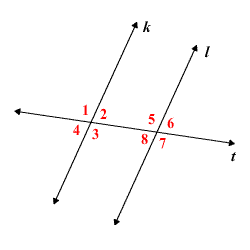# Alternate Exterior Angles Theorem

The Alternate Exterior Angles Theorem states that, when two parallel lines are cut by a transversal , the resulting alternate exterior angles are congruent .

So, in the figure below, if $k\parallel l$ , then

$\angle 1\cong \angle 7$ and $\angle 4\cong \angle 6$ .Proof.

Since $k\parallel l$ , by the Corresponding Angles Postulate ,

$\angle 1\cong \angle 5$ .

Also, by the Vertical Angles Theorem,

$\angle 5\cong \angle 7$ .

Then, by the Transitive Property of Congruence,

$\angle 1\cong \angle 7$ .

You can prove that $\angle 4$ and $\angle 6$ are congruent using the same method.

The converse of this theorem is also true; that is, if two lines $k$ and $l$ are cut by a transversal so that the alternate exterior angles are congruent, then $k\parallel l$ .